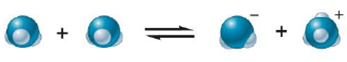Chapter 14, Problem 25Q

Chapter
Section
Textbook Problem

# Consider the autoionization of liquid ammonia:Label each of the species in the equation as an acid or a base and explain your answer.

Interpretation Introduction

Interpretation: In the given auto ionization equation of liquid ammonia, the given species as acid or base are to be labeled and explained.

Concept introduction: The remains of the acid molecule after the loss of proton is known as conjugate base.

When proton is transferred to the base, the product formed is known as conjugate acid.

Explanation

Explanation

To label: Each species in the given reaction as an acid or a base with proper explanation.

The labeled equation is,

NH3Acid+NH3BaseNH2ConjugateBase+NH4+ConjugateAcid

The first reactant species is NH3 . It is an acid.

The second reactant species is also NH3 . It is a base.

The product species that have positive charge is NH4+ . It is a conjugate acid.

The product species that have negative charge is NH2 . It is a conjugate base

### Still sussing out bartleby?

Check out a sample textbook solution.

See a sample solution

#### The Solution to Your Study Problems

Bartleby provides explanations to thousands of textbook problems written by our experts, many with advanced degrees!

Get Started

#### Find more solutions based on key concepts